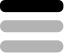Copywriting / Accounting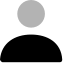Lau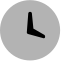9 months ago

## Turn equations into LaTex

#### Teaser

Turn any equation into LaTex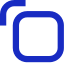#### Prompt Hint

The equation you want to transform#### Prompt

Learn more about the latest prompt: Turn equations into LaTex Get the details such as Turn any equation into LaTex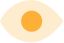345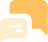614Try this prompt on ChatGPT